# ISEE Lower Level Math : How to find the perimeter of a square

## Example Questions

← Previous 1 3 4 5 6 7 8

### Example Question #1 : How To Find The Perimeter Of A Square

What is the perimeter of the square below?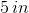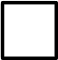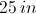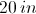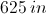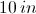Explanation:

To find the perimeter of a shape, take all of the outside measurements and add them together.  There are only 2 measurements showing. However, perimeter goes all the way around the square which has four equal sides.  Therefore, the measurement of the other sides must be the same and must be used to find the perimeter.

Take all four sides and add them together to find the perimeter of the square.  Since each side is equally 5 inches then the total of the sides (or perimeter of the square) would be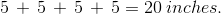### Example Question #1 : How To Find The Perimeter Of A Square

If the area of a square is 100 cm2 then what is the length of each side?  What is the perimeter of the square?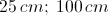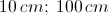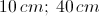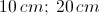Explanation:

The given area of the square was calculated by multiplying the measurement of one side of the square by the measurement of another side of the square (which are equal measurements).  Since the area was 100 cm then there was a number when multiplied to itself would be 100.  The number that makes this true is 10 as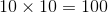It is now known that each side of the square has a length of 10 cm and to find the perimeter of the square the length of each side must be used to find the total length around the square.  Therefore, since each side is 10 cm then the perimeter can be found by adding all of the sides which would be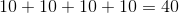The perimeter of the square would be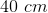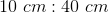### Example Question #1 : How To Find The Perimeter Of A Square

What is the perimeter of a square that has a side with a length of 12 cm.?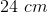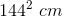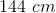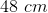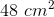Explanation:

To find the perimeter of a shape, you must add up the lengths of the sides. By definition, a square has 4 equal sides. So, you only need to know the length of one side of a square in order to know the lengths of all the sides of a square.

Since the length of one side of this square is 12 cm., you can either add the lengths of the four sides together or multiply the length of one side by 4.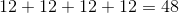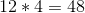The units used in this answer would be cm. — not cm.2 — because we are only find the length aroud the shape when solving for perimeter.

### Example Question #1 : How To Find The Perimeter Of A Square

The area of a square is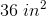.  What is the perimeter?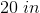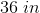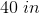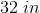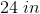Explanation:

The area of a square is given by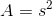. Here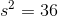, so take the square root of both sides to get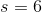.

The perimeter of a square is given by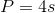. Substitute the side length obtained from the area equation to get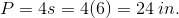### Example Question #2 : How To Find The Perimeter Of A Square

The area of a square is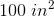. What is the perimeter?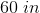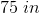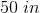Explanation:

The area of a square is given by, or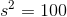.  Take the square root of both sides to get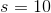.

The perimeter of a square is given by. Substitute the side length obtained from the area equation to get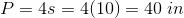.

### Example Question #1 : How To Find The Perimeter Of A Square

The area of a square is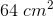.  What is the perimeter?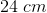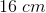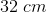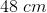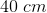Explanation:

The area of a square is given by, or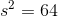. Take the square root of both sides to get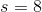.

The perimeter of a square is given by. Substitute the value obtained from the area equation to get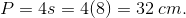### Example Question #1 : How To Find The Perimeter Of A Square

The area of a square is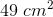. What is the perimeter?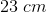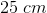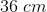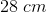Explanation:

The area of a square is given by, or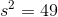. Take the square root of both sides to get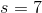.

The perimeter of a square is given by. Substitute the side length obtained from the area equation to get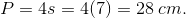### Example Question #1 : How To Find The Perimeter Of A Square

If the perimeter of a square is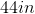What is the length of one of its sides?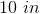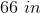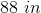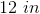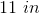Explanation:

The perimeter of a shape is equal to the sum of the lengths of each side. By definition, a square has 4 equal sides. As a result, you can divde the perimeter by 4 to get the length of one side: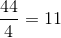Since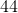divided byequals, then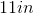is the length of one side of the square.

You can also check your answer by adding up the lengths of all the sides or by multiplying the length of one of the sides by. If you get, you are correct.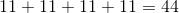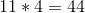### Example Question #10 : Squares

The area of a square is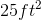. What is its perimeter?

20 feet

5 feet

3 feet

16 feet

25 feet

20 feet

Explanation:

The area of a square is calculated by multiplying one side by itself.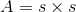If the area is 25, then the length of one side will be the square root of 25.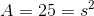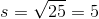The square root of 25 is 5, so each side must be 5 feet long.

If each of the four sides is 5 feet long, then the perimeter would be 20 feet because there are four sides total.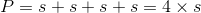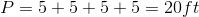### Example Question #1091 : Isee Lower Level (Grades 5 6) Mathematics Achievement

Which of the following is the perimeter of a square that has an area of 9 square inches?

6 inches

14 inches

12 inches

9 inches

12 inches

Explanation:

If a square has an area of 9 square inches, each side is 3 inches. This is because 3 inches times 3 inches is 9 inches. (The formula for the area of a square is length times hieght.)

Given that a square has 4 sides, we get the perimeter by multiplying 3 by 4, giving us a perimeter of 12 inches.

← Previous 1 3 4 5 6 7 8

### All ISEE Lower Level Math Resources